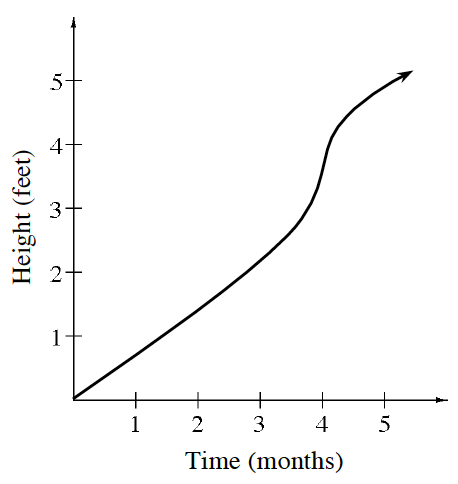### Home > APCALC > Chapter 2 > Lesson 2.3.3 > Problem2-127

2-127.

Fertilizer needs to be applied during the fastest growth of the plant. At right is the graph of the growth cycle of a flowering shrub.

1. Using complete sentences, write a detailed statement describing the growth of this shrub for $0\le t\le5$ months.

Pay attention to variance in the slope of the graph.

2. During what time interval should the plant be fertilized?

When does the fastest growth of the plant occur?

3. Approximately how fast is the shrub growing at $t = 3$? How did you get your answer?

Estimate the slope of the secant line at $t = 3$.

$\approx \frac{3}{4}\text{ feet/month}$

4. What is the shrub's average rate of growth over the complete growth cycle? How did you get your answer?

The average rate of growth is the slope of the secant line between $t = 0$ and $t = 5$.